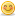1. Find the number....

Find the number using the following condition.

If you divided by 9, you will get the remainder 8
If you divided by 8, you will get the remainder 7
If you divided by 7, you will get the remainder 6
If you divided by 6, you will get the remainder 5
If you divided by 5, you will get the remainder 4
If you divided by 4, you will get the remainder 3
If you divided by 3, you will get the remainder 2
If you divided by 2, you will get the remainder 1

Clue: The number is less than 3000.

----------------------
sureshReply With Quote

2. Re: Find the number....

Hi suresh,

The number is 2519.Regards,
ElangoReply With Quote

3. Re: Find the number....

thanx Elango
is there any logic?
explain itReply With Quote

4. Re: Find the number....

It is very simple Sowji,

find LCM of 2,3,4...8 and 9 then subtract 1 from that number. that is itThanks,
JamesReply With Quote

5.Re: Find the number....

Hey can u please tell me whats the logic behind in taking L.cm's and subracting 1 from it..Reply With Quote

6. Re: Find the number....

HiReply With Quote

7. Re: Find the number....

I know how to make it

#include<stdio.h>
void main()
{
for(int q=1;q<30000;q++)
{
if((q%9==8)&&(q%8==7)&&(q%7==6)&&(q%6==5)&&(q%5==4)&&(q%4==3)&&(q%3==2)&&(q%2==1))
{
printf("%d",q);
break;
}
}
}Reply With Quote

8. Re: Find the number....

Ur Logic is correct Chayan.Reply With Quote

9. Re: Find the number....

good work .......Reply With Quote

10. Re: Find the number....Reply With Quote

11. Re: Find the number....Reply With QuotePosting Permissions

• You may not post new threads
• You may not post replies
• You may not post attachments
• You may not edit your posts
•